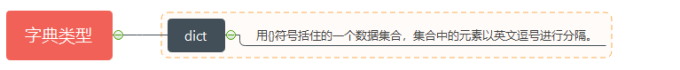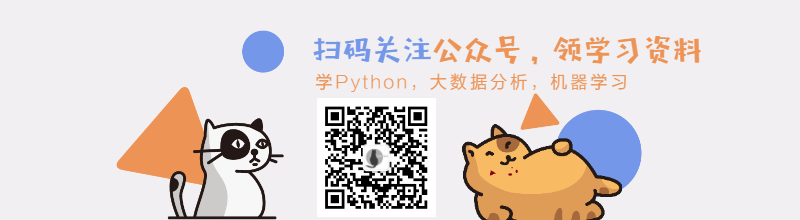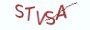Python基础教程

# 12.1.1 理解字典类型

Python中的字典类型是用{}符号括住的一个数据集合，集合中的元素以英文逗号进行分隔。`# 定义一个字典变量bookbook = {"第十二章-彻底掌握Python的字典类型": 210}`

# 12.1.2 字典的类型名

>>> book = {"第十二章-彻底掌握Python的字典类型": 210}
>>> type(book)
<class 'dict'>

# 12.1.3 字典的定义

(1) 对象定义法

class dict(object)
|  dict() -> new empty dictionary
|  dict(mapping) -> new dictionary initialized from a mapping object's
|      (key, value) pairs
|  dict(iterable) -> new dictionary initialized as if via:
|      d = {}
|      for k, v in iterable:
|          d[k] = v
|  dict(**kwargs) -> new dictionary initialized with the name=value pairs
|      in the keyword argument list.  For example:  dict(one=1, two=2)

(1) dict()
(2) dict(mapping)
(3) dict(iterable)
(4) dict(**kwargs)

1.dict()

```# __desc__ = 通过dict()构造一个空的字典
# 定义一个空的字典
book = dict()

# 空值在条件判断中会自动转换为布尔类型False

if book:
print("book is not empty")
else:
print("book is empty")```
2. dict(mapping)

```# __desc__ = 使用zip类型来定义一个mapping对象
# zip接受两个可迭代对象
# 元组是一种可迭代对象
# 迄今学过的可迭代对象有字符串，列表，元组

mapping = zip(("第十二章-彻底掌握Python的字典类型",), (210,))
# 使用mapping对象来构造字典对象
book = dict(mapping)
# book的输出为{"第十二章-彻底掌握Python的字典类型": 210}```
Python中的字典类型就是一种标准的mapping对象，我们可以在dict方法中传递一个字典对象来构造字典。

```# __desc__ = 使用字典类型来构造一个mapping对象
pair = {"第十二章-彻底掌握Python的字典类型": 210}
# 使用mapping对象来构造字典对象
book = dict(pair)
# book的输出为{"第十二章-彻底掌握Python的字典类型": 210}```
3. dict(iterable)

```# 1.列表中保存的是列表
[[key, value],[key,value]]
# 2.列表中保存的是元组
[(key, value),(key,value)]```

```# 1.元组中保存的是列表：
([key, value],[key,value])
# 2.元组中保存的是元组：
((key, value),(key,value))```

```# __desc__ = 通过可迭代对象来构造字典
book = dict([["第十二章-彻底掌握Python的字典类型", 20]])
# book的输出为{"第十二章-彻底掌握Python的字典类型": 210}

book = dict((("第十二章-彻底掌握Python的字典类型", 20),))
# book的输出为{"第十二章-彻底掌握Python的字典类型": 210}```
4.dict(**kwargs)

```# 在dict构造方法中，name=value即表示一个键值对
book = dict(name="108节课彻底学通Python")
# book的输出为{"name": "108节课彻底学通Python"}```
(2) 直接定义法

```# __desc__ = 直接通过{}来构造字典
# 构造一个空的字典
book = {}

# 构造一个有值的字典
book = {"name": "108节课彻底学通Python", "price": 0}```

# 12.1.4 在循环中遍历字典

```for name in dict:
pass```

```# __desc__ = 在for循环中遍历字典的键名
# 构造一个有值的字典
book = {"name": "108节课彻底学通Python", "author": "薯条老师"}
for name in book:
# 执行dict[name]的语法，可以访问字典的键值
print(name, book[name])```

# 12.1.6 知识要点

(1)Python中的字典类型是用{}符号括住的一个数据集合，集合中的元素以英文逗号进行分隔。
(2)字典中的元素，是以:分隔的键值对，键值对之间以逗号进行分隔
(3)字典的类型名是dict,可通过对象定义法和直接定义法来定义字典
(4)列表，元组是一种线性的序列结构。
(5)字典也是一种可迭代对象，可以在for循环中直接遍历出字典的键名

# 12.1.7 最具实力的小班培训

`(1) Python后端工程师高薪就业班，月薪11K-18K，免费领取课程大纲(2) Python爬虫工程师高薪就业班，年薪十五万，免费领取课程大纲(3) Java后端开发工程师高薪就业班，月薪11K-20K, 免费领取课程大纲(4) Python大数据分析，量化投资就业班，月薪12K-25K,免费领取课程大纲`## 注册账号